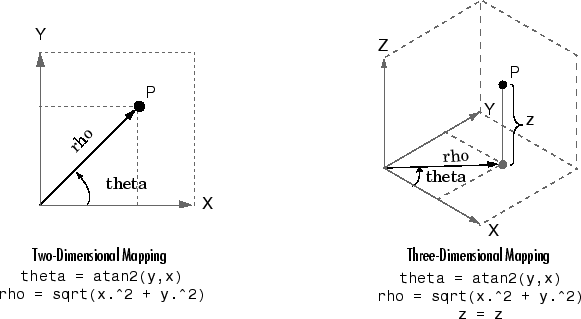# cart2pol

Transform Cartesian coordinates to polar or cylindrical

## Syntax

``````[theta,rho] = cart2pol(x,y)``````
``````[theta,rho,z] = cart2pol(x,y,z)``````

## Description

example

``````[theta,rho] = cart2pol(x,y)``` transforms corresponding elements of the two-dimensional Cartesian coordinate arrays `x` and `y` into polar coordinates `theta` and `rho`.```

example

``````[theta,rho,z] = cart2pol(x,y,z)``` transforms three-dimensional Cartesian coordinate arrays `x`, `y`, and `z` into cylindrical coordinates `theta`, `rho`, and `z`.```

## Examples

collapse all

Convert the Cartesian coordinates defined by corresponding entries in matrices `x` and `y` to polar coordinates `theta` and `rho`.

`x = [5 3.5355 0 -10]`
```x = 1×4 5.0000 3.5355 0 -10.0000 ```
`y = [0 3.5355 10 0]`
```y = 1×4 0 3.5355 10.0000 0 ```
`[theta,rho] = cart2pol(x,y)`
```theta = 1×4 0 0.7854 1.5708 3.1416 ```
```rho = 1×4 5.0000 5.0000 10.0000 10.0000 ```

Convert the three-dimensional Cartesian coordinates defined by corresponding entries in the matrices `x`, `y`, and `z` to cylindrical coordinates `theta`, `rho`, and `z`.

`x = [1 2.1213 0 -5]'`
```x = 4×1 1.0000 2.1213 0 -5.0000 ```
`y = [0 2.1213 4 0]'`
```y = 4×1 0 2.1213 4.0000 0 ```
`z = [7 8 9 10]'`
```z = 4×1 7 8 9 10 ```
`[theta,rho,z] = cart2pol(x,y,z)`
```theta = 4×1 0 0.7854 1.5708 3.1416 ```
```rho = 4×1 1.0000 3.0000 4.0000 5.0000 ```
```z = 4×1 7 8 9 10 ```

## Input Arguments

collapse all

Cartesian coordinates, specified as scalars, vectors, matrices, or multidimensional arrays. `x`, `y`, and `z` must be the same size, or have sizes that are compatible (for example, `x` is an `M`-by-`N` matrix, `y` is a scalar, and `z` is a scalar or `1`-by-`N` row vector). For more information, see Compatible Array Sizes for Basic Operations.

Data Types: `single` | `double`

## Output Arguments

collapse all

Angular coordinate, returned as an array. `theta` is the counterclockwise angle in the x-y plane measured in radians from the positive x-axis. The value of the angle is in the range ```[-pi pi]```.

Radial coordinate, returned as an array. `rho` is the distance from the origin to a point in the x-y plane.

Elevation coordinate, returned as an array. `z` is the height above the x-y plane.

## Algorithms

The mapping from two-dimensional Cartesian coordinates to polar coordinates, and from three-dimensional Cartesian coordinates to cylindrical coordinates is## Version History

Introduced before R2006a